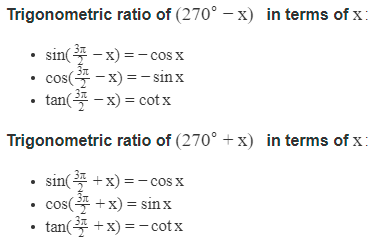Trigonometry Formulas for Class 11

# Trigonometry Formulas for Class 11

## Trigonometry Formulas for Class 11

If you are searching for all trigonometry formulas of Maths Class 11 at one place, then you are surely come to the right place. Maths-formula.com brings you all the important Maths formulas for class 11 to help you in your preparation for trigonometry class 11. These formulas are extremely important from the examinations’ point of view.

Maths is a subject where reasoning and logic are very important. Students should have a clear understanding of the underlying theories, concepts and formulas. They must understand that what are the different formulas and what they mean actually. Only then, they will be able to crack the mathematics questions asked in the examination. Here, we are providing all the formulas related to trigonometry for maths class 11.

## Trigonometry Formulas for Class 11

• Measurement of an angle: The measure of an angle is the amount of rotation from the initial side to the terminal side.
• Right angle: If the rotating ray starting from its initial position to final position, describes one quarter of a circle, then we say that the measure of the angle formed is a right angle.
• If in a circle of radius r, an arc of length l subtends an angle of θ radians, then l = rθ.
• Radian measure = π/180 × Degree measure
• Degree measure = 180/π × Radian measure

## Trigonometric Identities

• sin2 θ + cos2 θ = 1 ;       sin2 θ = 1 – cos2 θ ;           cos2 θ = 1 – sin2

sec2 θ – tan2 θ = 1 ;             sec2 θ = 1 + tan2 θ ;         tan2 θ = sec2 θ – 1

cosec2 θ – cot2 θ = 1 ;         cosec2 θ = 1 + cot2 θ ;      cot2 θ = cosec2 θ – 1Trigonometric ratio of (360 − x) in terms of x:

• cos (2π − x) = cos x
• sin (2π − x) = −sin x
• tan (2π − x) = −tan x

Trigonometric ratio of (360 + x) in terms of x:

• cos (2π + x) = cos x
• sin (2π + x) = sin x
• tan (2π + x) = tan x
• cos (2nπ + x) = cos x
• sin (2nπ + x) = sin x
• tan (2nπ + x) = tan x

Sum Formulae:

## Transformation Formulae

Product Formulae (on the basis of L.H.S.) or A-B formulae:

• 2 sin x cos y = sin (x + y) + sin (x − y)
• 2 cos x sin y = sin (x + y) – sin (x − y)
• 2 cos x cos y = cos (x + y) + cos (x − y)
• 2 sin x sin y = cos (x − y) – cos (x + y)

Sum and Difference Formulae (on the basis of L.H.S.) or C-D formulae:

## Trigonometric Equations

• Principle Solutions: The solutions of a trigonometric equation, for which 0 ≤ x < 2π are called the principle solutions.
• General Solutions: The solution, consisting of all possible solutions of a trigonometric equation is called its general solutions.

Some General Solutions:

• sin x = 0 gives x = nπ, where n  Z
• cos x = 0 gives x = (2n + 1)π/2, where n  Z
• tan x = 0 gives x = nπ
• cot x = 0 gives x = (2n + 1)π/2
• sec x = 0 gives no solution
• cosec x = 0 gives no solution
• sin x = sin y gives x = nπ + (−1)ny
• cos x = cos y gives x = 2nπ ± y, where n  Z
• tan x = tan y gives x = nπ + y, where n  Z
• sin 2x = sin 2y gives x = nπ ± y
• cos 2x = cos 2y gives x = nπ ± y
• tan 2x = tan 2y gives x = nπ ± y